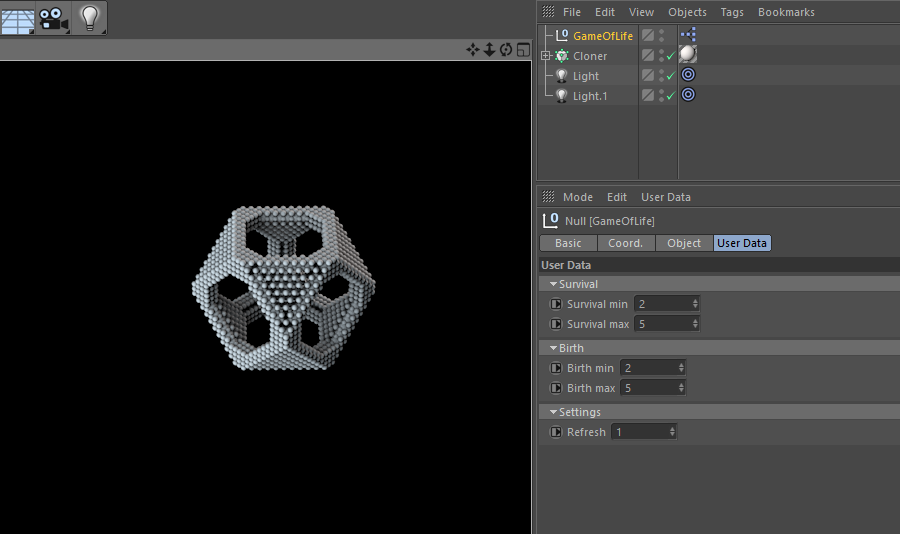## 26 January 2018

### [C4D Script] Random Vertex Color

RandomVertexColor is a python script for Cinema R18 and higher, which allows you to assign random color to connected vertices using the new Vertex Color Tag. I did this script very quickly, the implementation may be a little "naive". I'll try to make some optimizations next time.

This script will use the vertex color tag associated with the selected object, otherwise a new one is created. Afterwards, a random color is applied on every connected vertices chunk

### How to use ?

• Execute RandomVertexColor script by choosing it from the script menu or pressing the Execute button if you use the script manager```# -*- coding: utf-8 -*-

# Author: safina3d
# Website: safina3d.blogspot.com
# Version: 1.1
# Description: Assign random color to connected vertices using  Vertex Color Tag

import c4d
from c4d import utils, Vector, BaseContainer
from random import randint

def get_random_color():
""" Return a random color as c4d.Vector """

def get_random_value():
""" Return a random value between 0.0 and 1.0 """
return randint(0, 255) / 256.0

return Vector(get_random_value(), get_random_value(), get_random_value())

def get_connected_polygons(obj, remaining_polygons):
# type: (PolygonObject, List[int]) -> List[int]
bs = obj.GetPolygonS()
bs.DeselectAll()
bs.Select(remaining_polygons)
utils.SendModelingCommand(command=c4d.MCOMMAND_SELECTCONNECTED,
list=[obj],
mode=c4d.MODELINGCOMMANDMODE_POLYGONSELECTION,
bc=BaseContainer(),
doc=doc)
result = []
for polygon_index in remaining_polygons:
if bs.IsSelected(polygon_index):
result.append(polygon_index)

return result

def polygons_to_vertices(op, polygon_list):
# type: (BaseObject, List[int]) -> List[int]
vertices = []
for polygon_index in polygon_list:
pts = op.GetPolygon(polygon_index)
vertices += [pts.a, pts.b, pts.c, pts.d]

return list(set(vertices))

def get_chunks(op, remaining_polygons):
# type: (BaseObject, List[int]) -> List[List[int]]
result = []
while len(remaining_polygons) > 0:
connected_polygons = get_connected_polygons(op, remaining_polygons)
result.append(polygons_to_vertices(op, connected_polygons))
remaining_polygons = list(set(remaining_polygons).difference(connected_polygons))

return result

def main():
if op is None:
return

if op.GetType() != c4d.OBJECT_POLYGON:
return

vertex_color_tag = op.GetTag(c4d.Tvertexcolor)
if vertex_color_tag is None:
vertex_color_tag = c4d.VariableTag(c4d.Tvertexcolor, op.GetPointCount())
op.InsertTag(vertex_color_tag)

doc.SetActiveTag(vertex_color_tag)

remaining_polygons = list(range(op.GetPolygonCount()))

chunks = get_chunks(op, remaining_polygons)
for chunk in chunks:
color = get_random_color()
for vertex in chunk:
c4d.VertexColorTag.SetColor(data, None, None, vertex, color)

if __name__ == '__main__':
main()

```

## 01 January 2018

### [C4D Script] Game of life

Another programming classic : Game Of Life (Cellular Automata family).

Game of life is not a game and has nothing to do with life. but where it gets interesting, is in the fact that you can build complexe patterns whith different behaviours only with very simple rules.

The original Game of life rules (for a 2D space) are:

• Each cell with one or no neighbors dies, as if by solitude.
• Each cell with more than three neighbors dies, as if by overpopulation.
• Each cell with two or three neighbors survives.
• Any dead cell with exactly three live neighbours becomes a live cell, as if by reproduction.

In this script (Xpresso python node) I tried to create 3D version of Game of life with the possibility to have dynamic rules. You can start with some rules and change them right in the middle of your simulation.

It's a is time based animation. Every frame, new calculations are done and there is no bounderies for your final shape. as far as your rules allows expansion of your pattern and your PC can handle it :)

To create your own simulation, you have to :

1. Define a set of starting points (see fill_grid method in source code)
2. Set up your simulation rules
3. Press play button to start simulation### Source code

``````
import c4d
from c4d import documents, Vector
from collections import defaultdict

class MultidimensionalDictionary:

def __init__(self):
self.cells = defaultdict(lambda: defaultdict(dict))

def get_value(self, i, j, k):
if self.cells.has_key(i) and self.cells[i].has_key(j) and self.cells[i][j].has_key(k):
return self.cells[i][j][k]
return None

def set_value(self, i, j, k, value):
self.cells[i][j][k] = value
return self

def for_each(self, fn):
for ikey, ivalue in self.cells.items():
for jkey, jvalue in ivalue.items():
for kkey, kvalue in jvalue.items():
fn(kvalue, ikey, jkey, kkey)

class Helper:

@staticmethod
def create_dict():
return defaultdict(lambda: defaultdict(dict))

@staticmethod
def for_each(array, fn):
for value in array:
fn(value)

@staticmethod
def loop_around(i, j, k, fn):
for a in xrange(i - 1, i + 2):
for b in xrange(j - 1, j + 2):
for c in xrange(k - 1, k + 2):
if (a, b, c) != (i, j, k):
fn(a, b, c)

@staticmethod
def fill_grid(generation):
"""
"""
init_points = [(-1, 0, 0), (1, 0, 0), (0, 0, 1), (0, 0, -1), (0, 1, 0), (0, -1, 0)]

for p in init_points:
generation.grid.set_value(p, p, p, Cell())

class Cell:

def __init__(self, age=1):
self.age = age

class Generation:

def __init__(self):
self.grid = MultidimensionalDictionary()

def get_cells_count_around(self, i, j, k):
counter = 0
for a in xrange(i - 1, i + 2):
for b in xrange(j - 1, j + 2):
for c in xrange(k - 1, k + 2):
if self.grid.get_value(a, b, c):
counter += 1

return counter - 1 if self.grid.get_value(i, j, k) else counter

def get_next_generation(self):
""" Get next cells generation """

new_generation = Generation()

def birth(i, j, k):
""" Checks if a new cell can be born """
current_cell = self.grid.get_value(i, j, k)
if current_cell is None:
count = self.get_cells_count_around(i, j, k)
if birth_min <= count <= birth_max:
new_generation.grid.set_value(i, j, k, Cell())

def update_cell(selected_cell, i, j, k):
""" Checks if the current cell can survive """
count = self.get_cells_count_around(i, j, k)
if survival_min <= count <= survival_max:
selected_cell.age += 1
new_generation.grid.set_value(i, j, k, selected_cell)
Helper.loop_around(i, j, k, birth)

self.grid.for_each(update_cell)
return new_generation

class C4dRender:

SCALE_COEF = 1
OLDEST_CELL_AGE = 0

def __init__(self):
self.generation = Generation()
self.doc = documents.GetActiveDocument()
self.tp = self.doc.GetParticleSystem()
self.root_group = self.tp.GetRootGroup()

Helper.fill_grid(self.generation)

def update(self):
self.tp.FreeAllParticles()
self.generation.grid.for_each(self.render)
self.generation = self.generation.get_next_generation()

def render(self, cell, i, j, k):
C4dRender.OLDEST_CELL_AGE = cell.age if cell.age > C4dRender.OLDEST_CELL_AGE else C4dRender.OLDEST_CELL_AGE
# drawing particle
p = self.tp.AllocParticle()
self.tp.SetGroup(p, self.root_group)
self.tp.SetPosition(p, Vector(i, j, k) * C4dRender.SCALE_COEF)
self.tp.SetSize(p, cell.age * 0.5)
self.tp.SetColor(p, Vector(c4d.utils.RangeMap(cell.age, 0, C4dRender.OLDEST_CELL_AGE, 0, 1, True), 0.2, 0.4))

instance = C4dRender()

def main():

if frame % refresh == 0:
instance.update()
``````